Next: Non normalized Redundancy Up: suppl_html Previous: Summary of MI levels

## Redundancy in simultaneously recorded units

A frequently used measure of synergy and redundancy in the literature is the difference between the information conveyed by two cells together and that conveyed by the two cells considered individually,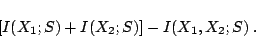(5)

This synergy-redundancy measure can be rewritten as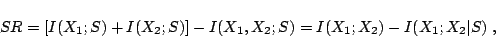(6)

and can be therefore interpreted as being the difference of two non-negative terms. The first,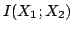, measures between-stimuli correlations and quantifies the stimulus induced redundancy; and the second,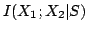, measures within-stimulus correlations and roughly corresponds to synergy.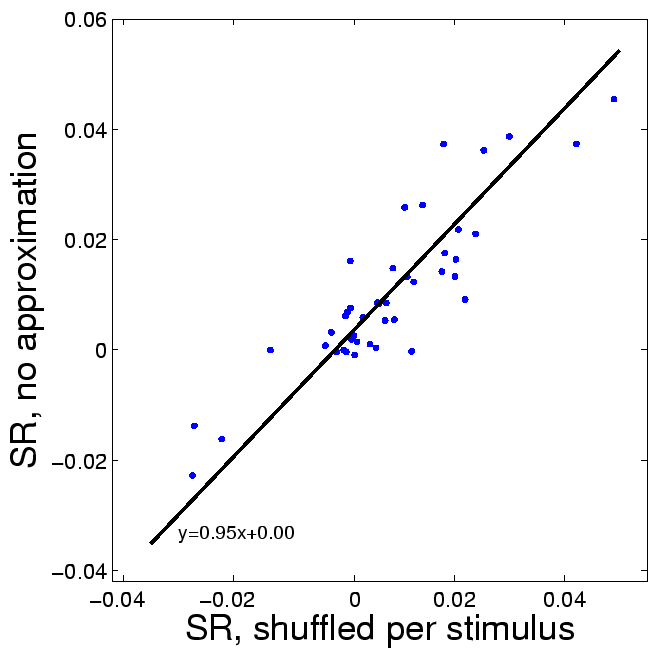We measured redundancy and synergy for a subset of cells that were recorded simultaneously (39 A1 cells forming 49 simultaneously recorded pairs) using a large number of presentation of each stimulus (100 repeats per stimulus). This number of repeats allowed reliable estimation of both the non conditioned informationand of the stimulus-conditioned informationin pairs of cells. To estimate the magnitude of the last term, we calculated SR, and then calculated it again after a random shuffling of the responses within each stimulus. In the limit of infinite data this shuffling should set the conditional information to zero, but finite sampling effects lead to distribution dependent biases . Figure S1 is a scatter plot of the stimulus-conditioned information estimated with and without shuffling, showing that the two are almost identical: the bi-directional regression line (measured as the first principal component) has a slope of 0.95, and the intercept is 0. Therefore, in this data, stimulus dependent correlations are negligible, and the SR measure can be well approximated usingunder the conditional independence approximation.

The use of the conditional independence approximation has considerable practical advantages. Computing the full standard synergy-redundancy value requires to estimate the full joint distributions of all stimuli and responses of two or more neurons. This requires a substantially larger number of repeats of each stimulus than is usually done for studying the responses of single neurons. For example, with only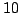stimuli, estimating redundancy in three neurons with dynamical range ofvalues requires at least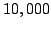stimulus repeats, which are extremely hard to obtain in electrophysiological experiments. With the conditional independence approximation, the number of parameters that are required to compute the MI depends linearly, rather than exponentially, on the number of neurons, making MI estimation manageable. Furthermore, using the conditional-independence approximation allowed us to increase our sample using neuronal pairs and triplets that were not recorded simultaneously. Finally, such conditional independence approximations are prominent in learning multivariate distributions and often allow more reliable estimation with limited samples . The lesson learned from this field is that when distributions are estimated from examples, better accuracies are often obtained when applying �incorrect� independence approximations, since these allow more reliable estimation of the joint distribution. In fact, the conditional independence approximation has been used previously in neural redundancy calculations, for precisely the above reasons, for example by .Next: Non normalized Redundancy Up: suppl_html Previous: Summary of MI levels
Gal Chechik 2006-07-19